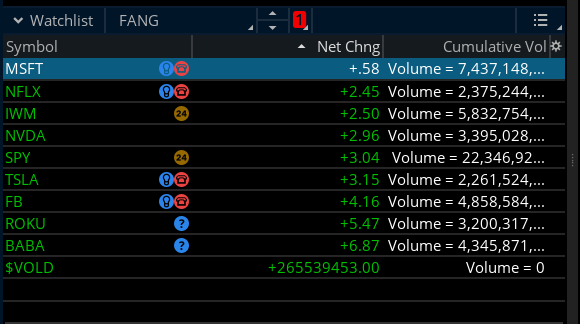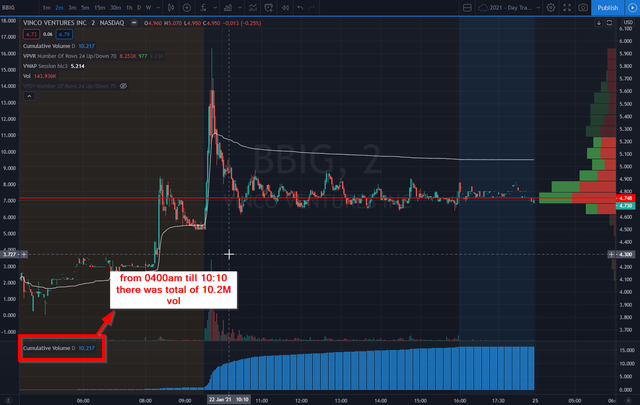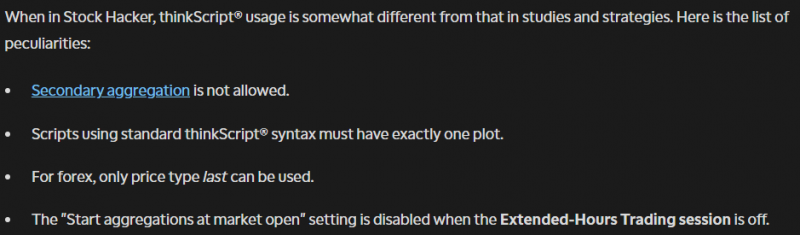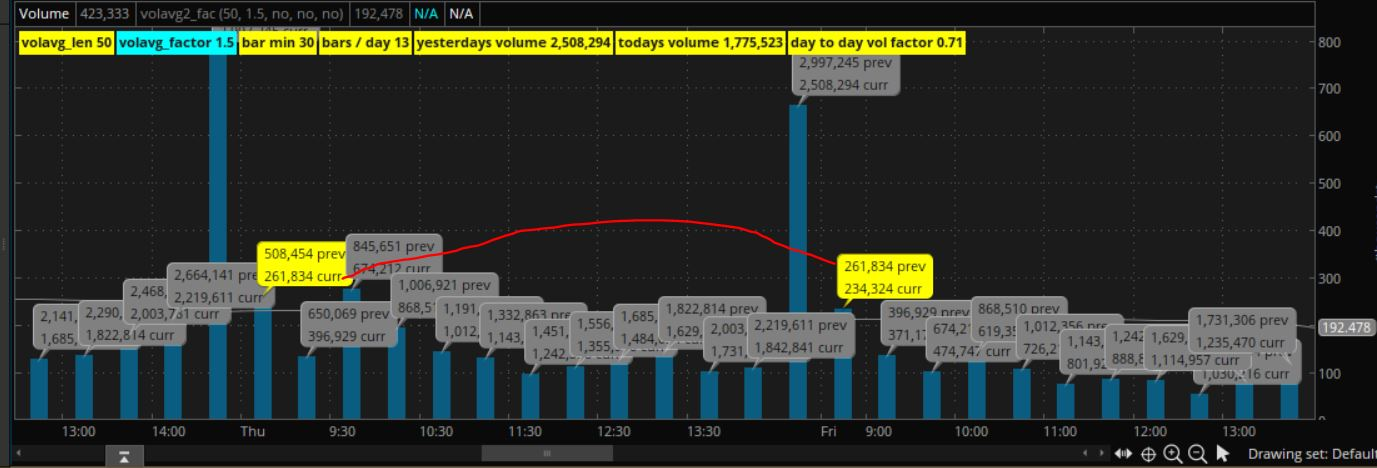# Cumulative Volume Chart, Watchlists, Scan, Label for ThinkorSwim

#### BenTen

Staff member
Staff
VIP
Here is a watchlist column that shows cumulative volume during an active period.Code:
``````# Archive Name: Volume - Cumulative Volume During An Active Period_Mobius
#  Archive Section: Watchlists
# Suggested Tos Name: Volume_CumulativeActivePeriod_Mobius

# Cumulative Volume During An Active Period
# Mobius
# Chat Room Request 05.01.2018

input startTime = 0930;
input endTime = 1600;

def Active = SecondsFromTime(startTime) >= 0 and
SecondsTillTime(endTime) >= 0;
def v = volume;
def cumulative = if Active and !Active
then v
else if Active
then cumulative + v
else cumulative;
addLabel(1, "Volume = " + cumulative, color.white);``````

Credits:

•Hello!I was wondering if there is any way you can get a value on watchlist that shows me the cumulative volume for a specific time period?
I have tried to change this to work in a watchlist column instead of what it is showing on chart.

for example:

input startTime = 1000;
input endTime = 1030;

def Active = SecondsFromTime(startTime) >= 0 and
SecondsTillTime(endTime) >= 0;
def v = volume;
def cumulative = if Active and !Active
then v
else if Active
then cumulative + v
else cumulative;I need to get a plot in there somehow, but everything i have done so far has not gotten me the result i am looking for.
Do i need to rethink the script or is it something else i need to consider to make this work?

thank you

Try replacing the last line:
Code:
``addLabel(1, cumulative);``
with this:
Code:
``plot x = cumulative;``

Just make sure the aggregation is set to 1 minute

•Gamma
Thank you for a quick answer!

•Len20
Hello good people,

I would like to ask if anyone knows if there's such a script for cumulative volume that calculates the cumulative volume of the day?Thank you!!

Hello good people,

I would like to ask if anyone knows if there's such a script for cumulative volume that calculates the cumulative volume of the day?Thank you!!
VolumeProfile in studies

I came across an indicator regarding Intraday Cumulative Volume, I think it makes sense. I put it into a scanner but it doesn't work, please take a look. Thanks

The code

declare lower;

def period = GetAggregationPeriod();
def minutesPerBar = period / 60000;
def minutesPerDay = 60 * 6.5; # 6.5 hours of standard trading session
def bpd = RoundUp( minutesPerDay / minutesPerBar, 0); # bars per day

def daycnt = 20; # Number of days

# This is the cumulative volume for the current day
def day = GetDay();
def cumVolume =
if day != day then volume
else cumVolume + volume
;

# average of cumulative volume at this time of day for the last 20 trading days
def avgCumVolume = (cumVolume[(bpd * 1)] + cumVolume[(bpd * 2)] + cumVolume[(bpd * 3)] + cumVolume[(bpd * 4)] + cumVolume[(bpd * 5)] + cumVolume[(bpd * 6)] + cumVolume[(bpd * 7)] + cumVolume[(bpd * 8)] + cumVolume[(bpd * 9)] + cumVolume[(bpd * 10)] + cumVolume[(bpd * 11)] + cumVolume[(bpd * 12)] + cumVolume[(bpd * 13)] + cumVolume[(bpd * 14)] + cumVolume[(bpd * 15)] + cumVolume[(bpd * 16)] + cumVolume[(bpd * 17)] + cumVolume[(bpd * 18)] + cumVolume[(bpd * 19)] + cumVolume[(bpd * 20)]) / daycnt;

plot RVol = Round(if IsNaN(cumVolume) then 0 else cumVolume / avgCumVolume, 3);

RVol.SetPaintingStrategy(PaintingStrategy.HISTOGRAM);

The screenshot

I need some help...Since volume at the beginning of the day tends to be higher...I want to create a script that allows me to Create my own weighting system for volume....Say 930 to 1030 is weighted at 70% of other time points.

My Ask: Is there a way to plot cumulative volume STARTING with designated time period...

Below I used the seconds from 0930 then divided by 60 so it would plot the MINUTE cumulative vale.....I must be overlooking something...Not getting the results

def len = volume(period = AggregationPeriod.DAY) / ((SecondsFromTime(fromTime = 930)/60));
plot vol = len;
vol.SetDefaultColor(color = color.red);

Secondary aggregations are not available in scans. I'm sure a quick search may point you to some of the more informative.

-mashume

Last edited by a moderator:
Secondary aggregations are not available in scans. I'm sure a quick search may point you to some of the more informative.

-mashume
Thanks, any way to modify the code to work around this problem here?

I need some help...Since volume at the beginning of the day tends to be higher...I want to create a script that allows me to Create my own weighting system for volume....Say 930 to 1030 is weighted at 70% of other time points.

My Ask: Is there a way to plot cumulative volume STARTING with designated time period...

Below I used the seconds from 0930 then divided by 60 so it would plot the MINUTE cumulative vale.....I must be overlooking something...Not getting the results

i am not sure what you are asking. maybe this will help.
this will define 3 time periods during a day. a number is assigned to each period. that number will be multiplied by the volume of each bar, in a period. a line is drawn on the volume chart, of those adjusted volume levels.

each day, dayvol will equal the total adjusted volume of the day.

Ruby:
``````# define 3 time periods
input t1_start = 0930;
input t1_end = 1030;
def t1 = if secondsfromTime(t1_start) >= 0 and secondstillTime(t1_end) > 0 then 1 else 0;

input t2_start = 1030;
input t2_end = 1300;
def t2 = if secondsfromTime(t2_start) >= 0 and secondstillTime(t2_end) > 0 then 1 else 0;

input t3_start = 1300;
input t3_end = 1600;
def t3 = if secondsfromTime(t3_start) >= 0 and secondstillTime(t3_end) > 0 then 1 else 0;

# apply different multipliers to volume, during 3 different time periods
def vol_factor;
if t1 then {
# 9:30 to 10:30  70%
vol_factor = 0.7;
} else if t2 then {
# 10:30 to 1:00  100%
vol_factor = 1;
} else if t3 then {
# 1:00 to 4:00  100%
vol_factor = 1;
} else {
# other times  100%
vol_factor = 1;
}

plot volx = round(volume * vol_factor, 0);
volx.setdefaultcolor(color.yellow);

# add up volume each day
def dayofwk = GetDayofWeek(GetYYYYMMDD() );
def diffday = (dayofwk <> dayofwk );
def dayvol = if diffday then volx else dayvol + volx;

# show total adjusted volume, under the volume bars, in units of millions
plot v_mil = round(dayvol/1000000, 1);
v_mil.SetPaintingStrategy(PaintingStrategy.VALUES_BELOW);
v_mil.setdefaultcolor(color.yellow);
#``````

Last edited:
••andreythedon and MerryDay
Thanks, any way to modify the code to work around this problem here?
The scanner is built on the premise that you are scanning one timeframe.

The Peculiarities Of Secondary AggregationsThere is no work-around to the limitation of no secondary aggregations can be used in the TOS Scan Hacker

@halcyonguy

Could you explain in basic terms what "def diffday = (dayofwk <> dayofwk );" means...I imagine it's trying to define that the current day is NOT EQUAL to a day ago??

@halcyonguy

Could you explain in basic terms what "def diffday = (dayofwk <> dayofwk );" means...I imagine it's trying to define that the current day is NOT EQUAL to a day ago??
correct.

diffday will be true on the first bar of each day. because the previous bar value of dayofwk, the  , will have a different value than the current bar.

•andreythedon
Would like to see what you come up with. I think seeing cumulative TICK starting and endign with each half hour or hour during the day would be helpful to see.

For the above code, seems it doesn't work properly, like the calculation is not started at 6:30 am, don't know if something is related to the settings, could you please take a look into it?

Thanks

Last edited by a moderator:
I would like to see that too

Hey I have played with thinkscript a bit, most of my experience is in a normal language on indexed dataframes, not rolling data like thinkorswim uses. Anyway, I am looking to create labels on a chart that show how much volume a security had yesterday, at today's time. So, if the market has been open for 2 hours, I would like to create a label with the accrued volume of yesterday up until 2 hours in. I primarily daytrade on the 5min chart so I started this script using the "fold" function, which I am not super familar with, but I think it is very similar to a traditional for loop? Anyway, here is my code for this on a 5min chart. The idea is that there are 80 5min periods in a day of trading so I would take the current barnumber() on my chart, and add it to 80, which should bring me to the start of yesterday, and 80 periods behind the current period should be the same period from the current day. When I apply this to a label, the label will not appear, doesnt even give me a "NaN" or anything. I am guessing I am using the fucntion wrong or something. Any help would be much appreciated.

Thanks

Code:
``````def bar = barNumber();
def yesterday_start = barnumber() + 80;
def yesterday_stop = 80;

def v = fold index = yesterday_start to 80 with v = 0 do v + volume[index];``````

Hey I have played with thinkscript a bit, most of my experience is in a normal language on indexed dataframes, not rolling data like thinkorswim uses. Anyway, I am looking to create labels on a chart that show how much volume a security had yesterday, at today's time. So, if the market has been open for 2 hours, I would like to create a label with the accrued volume of yesterday up until 2 hours in. I primarily daytrade on the 5min chart so I started this script using the "fold" function, which I am not super familar with, but I think it is very similar to a traditional for loop? Anyway, here is my code for this on a 5min chart. The idea is that there are 80 5min periods in a day of trading so I would take the current barnumber() on my chart, and add it to 80, which should bring me to the start of yesterday, and 80 periods behind the current period should be the same period from the current day. When I apply this to a label, the label will not appear, doesnt even give me a "NaN" or anything. I am guessing I am using the fucntion wrong or something. Any help would be much appreciated.

Thanks

Code:
``````def bar = barNumber();
def yesterday_start = barnumber() + 80;
def yesterday_stop = 80;

def v = fold index = yesterday_start to 80 with v = 0 do v + volume[index];``````

first, let me say good job on trying to do it and tackling the use of a fold. Fold loops are tricky and can take a while to get figured out, but it looks like you've got the basic set up pretty close.

1. There are 6.5 hours in a trading day, or 390 minutes.
So there will be 78 , 5 minute bars. At the closing bell that bar is not counted. that is an after hours.

2. Most of the time looking back X number of bars will work. but occasionally on some stocks there is a time period with no trades, so there won't be the usual quantity of bars per the day, and that will throw off studies like this.

3. In your fold loop the start number is bigger than the end number, so it won't even run through one iteration..
In a fold, think of it as running through a series of offset numbers. so maybe start at 0 or 1 to some bigger number.

4. When working in fold loops try to use getvalue to read data instead of the normal offset.
do v + volume[index];
use this,
do v + getvalue(volume, index);

------------------------------

This version will have issues with missing bars during the day, ( no volume or halts), but should get you some numbers.
add up volume during the day, for each day.

i added code to calculate how many bars in a day, so it will work on any time, less than a day.
the chart doesn't have to be 5 minutes.

it looks back x bars and reads a volume total from yesterday.

Ruby:
``````# prev_day_vol_0

# Getting Yesterdays cumulative volume at today's time

# for charts with a period less than a day.
# get the chart agg time and convert to minutes. then calculate how many bars are in the trading day, on the chart.
def chartagg = GetAggregationPeriod();
def chartmin = chartagg / (1000 * 60);
# 6.5 hours in a normal trading day, 390 minutes.
def daybars = 390/chartmin;

addlabel(1, "bar min " + chartmin, color.yellow);
addlabel(1, "bars / day " + daybars, color.yellow);

# 78 for 5min bars
# def daybars = 78;

def isstart = If secondsfromtime(930) == 0 then 1 else 0;
def dayvol = If isstart then volume else if secondsfromtime(930) > 0 and secondstilltime(1600) > 0 then dayvol + volume else dayvol;
#def prevdayvol = dayvol[daybars];
def prevdayvol = getvalue(dayvol, daybars);

addlabel(1, "yesterdays volume " + prevdayvol, color.yellow);
addlabel(1, "todays volume " + dayvol, color.yellow);
addlabel(1, "day to day vol factor " + round(dayvol/prevdayvol,2), color.yellow);

input test1_vol_bubbles = no;
prevdayvol + " prev\n" +
dayvol + " curr"
, (if isstart then color.yellow else color.gray), yes);
#``````

with test bubbles turned on , on a 30min chart
shows previous day accrued volume over current day accrued volumegetvalue has a 3rd parameter that should be used, max offset, to prevent bad code from causing endless loops.
https://tlc.thinkorswim.com/center/reference/thinkScript/Functions/Others/GetValue

Well thank you! I appreciate you finishing/fixing it. I figured there was something wrong with how I was using the function. I was thinking of the index from oldest to most current instead of smallest to biggest, since the larger the index the older the value with think script. That is awesome! Thank you very much

•halcyonguy

### Not the exact question you're looking for?

87k+ Posts
267 Online## The Market Trading Game Changer

Join 2,500+ subscribers inside the useThinkScript VIP Membership Club
• Exclusive indicators
• Proven strategies & setups
• Private Discord community
• Exclusive members-only content
• 1 full year of unlimited support

What is useThinkScript?

useThinkScript is the #1 community of stock market investors using indicators and other tools to power their trading strategies. Traders of all skill levels use our forums to learn about scripting and indicators, help each other, and discover new ways to gain an edge in the markets.

How do I get started?

We get it. Our forum can be intimidating, if not overwhelming. With thousands of topics, tens of thousands of posts, our community has created an incredibly deep knowledge base for stock traders. No one can ever exhaust every resource provided on our site.

If you are new, or just looking for guidance, here are some helpful links to get you started.

What are the benefits of VIP Membership?